# Permutations without repetition

Find out how many different ways you can choose k items from n items set. With/without repetition, with/without order.

(n)
(k)

39916800

# A bit of theory - foundation of combinatorics

## Variations

A variation of the k-th class of n elements is an ordered k-element group formed from a set of n elements. The elements are not repeated and depend on the order of the group's elements (therefore arranged).

The number of variations can be easily calculated using the combinatorial rule of product. For example, if we have the set n = 5 numbers 1,2,3,4,5 and we have to make third-class variations, their V3 (5) = 5 * 4 * 3 = 60.
${V}_{k}\left(n\right)=n\left(n-1\right)\left(n-2\right)\mathrm{.}\mathrm{.}\mathrm{.}\left(n-k+1\right)=\frac{n!}{\left(n-k\right)!}$
n! we call the factorial of the number n, which is the product of the first n natural numbers. The notation with the factorial is only clearer, equivalent. For calculations, it is fully sufficient to use the procedure resulting from the combinatorial rule of product.

## Permutations

The permutation is a synonymous name for a variation of the nth class of n-elements. It is thus any n-element ordered group formed of n-elements. The elements are not repeated and depend on the order of the elements in the group.
$P\left(n\right)=n\left(n-1\right)\left(n-2\right)\mathrm{.}\mathrm{.}\mathrm{.}1=n!$
A typical example is: We have 4 books, and in how many ways can we arrange them side by side on a shelf?

## Variations with repetition

A variation of the k-th class of n elements is an ordered k-element group formed of a set of n elements, wherein the elements can be repeated and depends on their order. A typical example is the formation of numbers from the numbers 2,3,4,5, and finding their number. We calculate their number according to the combinatorial rule of the product:
${V}_{k}^{\mathrm{\prime }}\left(n\right)=n\cdot n\cdot n\cdot n\mathrm{.}\mathrm{.}\mathrm{.}n={n}^{k}$

## Permutations with repeat

A repeating permutation is an arranged k-element group of n-elements, with some elements repeating in a group. Repeating some (or all in a group) reduces the number of such repeating permutations.
${P}_{{k}_{1}{k}_{2}{k}_{3}\mathrm{.}\mathrm{.}\mathrm{.}{k}_{m}}^{\mathrm{\prime }}\left(n\right)=\frac{n!}{{k}_{1}!{k}_{2}!{k}_{3}!\mathrm{.}\mathrm{.}\mathrm{.}{k}_{m}!}$
A typical example is to find out how many seven-digit numbers formed from the numbers 2,2,2, 6,6,6,6.

## Combinations

A combination of a k-th class of n elements is an unordered k-element group formed from a set of n elements. The elements are not repeated, and it does not matter the order of the group's elements. In mathematics, disordered groups are called sets and subsets. Their number is a combination number and is calculated as follows:
${C}_{k}\left(n\right)=\left(\genfrac{}{}{0px}{}{n}{k}\right)=\frac{n!}{k!\left(n-k\right)!}$
A typical example of combinations is that we have 15 students and we have to choose three. How many will there be?

## Combinations with repeat

Here we select k element groups from n elements, regardless of the order, and the elements can be repeated. k is logically greater than n (otherwise, we would get ordinary combinations). Their count is:
${C}_{k}^{\mathrm{\prime }}\left(n\right)=\left(\genfrac{}{}{0px}{}{n+k-1}{k}\right)=\frac{\left(n+k-1\right)!}{k!\left(n-1\right)!}$
Explanation of the formula - the number of combinations with repetition is equal to the number of locations of n − 1 separators on n-1 + k places. A typical example is: we go to the store to buy 6 chocolates. They offer only 3 species. How many options do we have? k = 6, n = 3.

## Foundation of combinatorics in word problems

• Cards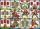How many ways can give away 32 playing cards to 7 player?
• ChordsHow many 4-tones chords (chord = at the same time sounding different tones) is possible to play within 7 tones?
• Soccer teams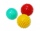Have to organize soccer teams. There are 3 age groups. How many different ways can you organize teams of ten for each age group? Is this a permutation or combination?
• CardsThe player gets 8 cards of 32. What is the probability that it gets a) all 4 aces b) at least 1 ace
• Football team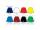The football team has black, purple, and orange shirts, blue and white shorts, and striped and gray socks. How many different outfits players may start?
• Divisible by fiveHow many different three-digit numbers divisible by five can we create from the digits 2, 4, 5? The digits can be repeated in the created number.
• Three digits number 2Find the number of all three-digit positive integers that can be put together from digits 1,2,3,4 and which are subject to the same time has the following conditions: on one positions is one of the numbers 1,3,4, on the place of hundreds 4 or 2.
• Honored students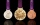Of the 25 students in the class, 10 are honored. How many ways can we choose 5 students from them, if there are to be exactly two honors between them?
• Win in raffle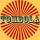The raffle tickets were sold 200, 5 of which were winning. What is the probability that Peter, who bought one ticket will win?
• CombinationsHow many different combinations of two-digit number divisible by 4 arises from the digits 3, 5 and 7?
• Covid-19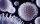Data showed that 22% of people in a small town was infected with the COVID-19 virus. A random sample of six residents from this town was selected. Find the probability that exactly two of these residents was infected.
• Karolína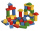Karolína chose 5 bodies from the kit - white, blue and gray cubes, a blue cylinder and a white triangular prism. How many different roof towers can be built one by one if all the blue bodies (cube and cylinder) are not placed on top of each other?
• Seagull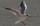Artificially created island in the shape of a circle with a radius of 50 m is overgrown with grass. The only exception is a landing area for helicopters in the shape of a rectangle measuring 15 m and 8 m. What is the probability that the flying seagull (w
• Three-digit numbersWe have digits 0,1,4,7 that cannot be repeated. How many three-digit numbers can we write from them? You can help by listing all the numbers.
• Tournament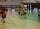Determine how many ways can be chosen two representatives from 34 students to school tournament.
• DiceWe throw 10 times a playing dice. What is the probability that the six will fall exactly 4 times?